Most Affordable JEE | NEET | 8,9,10 Preparation by Kota's Top IITian Doctor Faculties

# Oblique Projectile motion - Motion in Plane - eSaralHey, do you want to learn about the Oblique Projectile motion? If yes. Then keep reading.

## Oblique Projectile motion

Consider the motion of a bullet which is fired from a gun so that its initial velocity $\overrightarrow{\mathrm{u}}$ makes an angle $\theta$ with the horizontal direction. Let us take $X$ -axis along ground and Y-axis along vertical.

$\overrightarrow{\mathrm{u}}$ can be resolved as

$\mathrm{u}_{\mathrm{x}}=\mathrm{u} \cos \theta \quad$ (along horizontal)

$\& u_{y}=u \sin \theta$ (along vertical)

motion of bullet can be resolved into horizontal and vertical motion.

(i) In horizontal direction there is no acc. so it moves with constant velocity $v_{x}=u_{x}=u \cos \theta$

So distance traversed in time $t$ is $x=u_{x} t$ or  $t=\frac{x}{u \cos \theta}$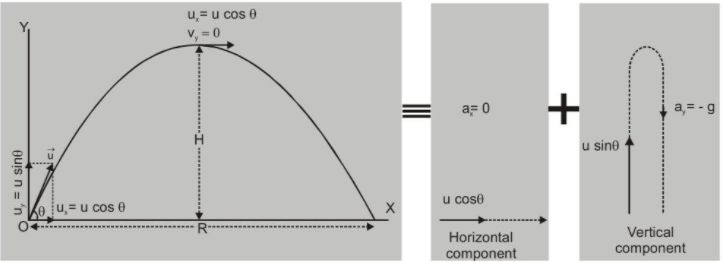The motion in the vertical direction is the same as that of a ball thrown upward with an initial velocity $\mathrm{u}_{y}=\mathrm{u} \sin \theta$ and $\mathrm{acc}=-\mathrm{g}$ (downward).

So at time $t$ vertical component of velocity $v_{y}=u_{y}-g t=u \sin \theta-g t$

Displacement along y direction

$y=(u \sin \theta) t-\frac{1}{2} g t^{2}$

Substituting the value of $t$ from eqn. (i) in eqn. (iii)

we get

$\quad y=(u \sin \theta)\left(\frac{x}{u \cos \theta}\right)-\frac{1}{2} g\left(\frac{x}{u \cos \theta}\right)^{2}$

or $\left.y=x \tan \theta-\frac{g}{2 u^{2} \cos ^{2} \theta} \cdot x^{2}\right]$ This is eqn. of parabola.

The trajectory of projectile is parabolic

The projectile will rise to maximum height $\mathrm{H}$ (where $\mathrm{v}_{x}=\mathrm{u} \cos \theta, \mathrm{v}_{y}=0$ ) and then move down again to reach the ground at a distance $\mathrm{R}$ from origin.

Setting $x=R$ and $y=0$ (since projectile reaches ground again)

$\mathrm{O}=\mathrm{R} \tan \theta-\frac{\mathrm{g}}{2 \mathrm{u}^{2} \cos ^{2} \theta} \cdot \mathrm{R}^{2}$

We get $R=\frac{2 u^{2} \cos ^{2} \theta}{g} \times \frac{\sin \theta}{\cos \theta}$

or $\mathrm{R}=\frac{2 \mathrm{u}^{2}}{\mathrm{~g}} \cdot \sin \theta \cos \theta$

or Range $R=\frac{u^{2} \sin 2 \theta}{g}$

If time for upward journey is t

at highest point $\quad v_{y}=0$ so $0=(u \sin \theta)-g t$

$\left(v_{y}=u_{y}-g t\right)$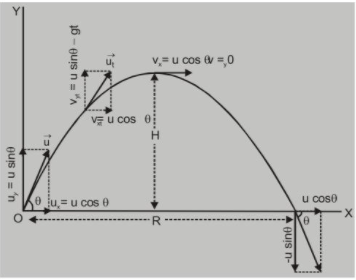or $\mathrm{t}=\frac{\mathrm{u} \sin \theta}{\mathrm{g}}$

$\mathrm{T}=2 \mathrm{t} \quad$ (it will take same time for downward journey)

$\therefore$

$T=\frac{2 u \sin \theta}{g}$ Time of flight

At the highest point $y=H$ and $v_{y}=0$ Maximum Height and Time of

Flight Depends on Vertical

Component of Initial Velocity So that $H=\frac{u_{y}^{2}}{2 g} \quad\left[v_{y}^{2}=u_{y}^{2}-2 g y\right]$

or $\mathrm{H}=\frac{\mathrm{u}^{2} \sin ^{2} \theta}{2 \mathrm{~g}}$ Maximum Height

we can also determine $R$ as follows

so $x=u_{x} t$

$R=u_{x} \cdot T$

$=(u \cos \theta)\left(\frac{2 u \sin \theta}{g}\right)$

or $\mathrm{R}=\frac{\mathrm{u}^{2} \sin 2 \theta}{\mathrm{g}}$

velocity at time t $\vec{v}_{t}=v_{x t} \hat{i}+v_{y t} \hat{j}$

$=(u \cos \theta) \hat{i}+(u \sin \theta-g t) \hat{j}$

$v=\sqrt{u^{2} \cos ^{2} \theta+(u \sin \theta-g t)^{2}}$

Note :

(i) Alternative $e q^{n}$, of trajectory $y=x \tan \theta\left(1-\frac{x}{R}\right)$ where $R=\frac{2 u^{2} \sin \theta \cos \theta}{g}$

(ii) Vertical component of velocity $v_{y}=0,$ when particle is at the highest point of trajectory.

(iii) Linear momentum at highest point = mu cos $\theta$ is in horizontal direction.

(iv) Vertical component of velocity is +ive when particle is moving up.

(v) Vertical component of velocity is -ive when particle is moving down.

(vi) Resultant velocity of particle at time $t \vee=\sqrt{v_{x}^{2}+v_{y}^{2}}$ at an angle $\phi=\tan ^{-1}\left(\frac{v_{y}}{v_{x}}\right) .$

(vii) Displacement from origin, $s=\sqrt{x^{2}+y^{2}}$

Special Points :

(1) The three basic equation of motion, i.e.

$v=u+a t$

$\mathrm{s}=\mathrm{ut}+\frac{1}{2} \mathrm{at}^{2} \quad \mathrm{v}^{2}=\mathrm{u}^{2}+2 \mathrm{as}$

For projectile motion give:

$T=\frac{2 u \sin \theta}{g} \quad R=\frac{u^{2} \sin 2 \theta}{g}$

$H=\frac{u^{2} \sin ^{2} \theta}{2 g}$

(2) In case of projectile motion,

The horizontal component of velocity (u $\cos \theta$ ), acceleration (g), and mechanical energy remain constant.

Speed, velocity, vertical component of velocity (u $\sin \theta)$, momentum, kinetic energy and potential energy all change. Velocity and K.E. are maximum at the point of projection, while minimum (but not zero) at the highest point.

(3) If angle of projection is changed from

then range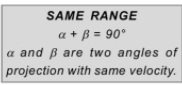$\theta-\theta=(90-\theta)$

$R=\frac{u^{2} \sin 2 \theta^{\prime}}{g}=\frac{u^{2} \sin 2(90-\theta)}{g}=\frac{u^{2} \sin 2 \theta}{g}=R$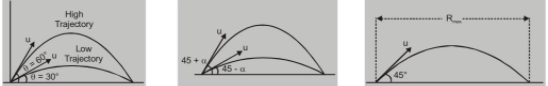So a projectile has same range for angles of projection $\theta$ and $(90-\theta)$

But has different time of flight (T), maximum height (H) \& trajectories

Range is also same for $\theta_{1}=45^{\circ}-\alpha$ and $\quad \theta_{2}=45^{\circ}+\alpha .\left[\right.$ equal $\left.\frac{u^{2} \cos 2 \alpha}{g}\right]$

(4) For maximum Range $\quad R=R_{\max } \Rightarrow 2 \theta=90^{\circ}$

$\text { for } \quad \theta=45^{\circ}$

$R_{\max }=\frac{u^{2}}{g} \quad\left[\right.$ For $\sin 2 \theta=1=\sin 90^{\circ}$ or $\left.\theta=45^{\circ}\right]$

When range is maximum $\Rightarrow$ Then maximum height reached

$\mathrm{H}=\frac{\mathrm{u}^{2} \sin ^{2} 45}{2 \mathrm{~g}}\left(\mathrm{When} \mathrm{R}_{\max }\right)$

or

$\mathrm{H}=\frac{\mathrm{u}^{2}}{4 \mathrm{~g}}$

hence maximum height reached (for $\left.R_{\max }\right) \quad H=\frac{R_{\max }}{4}$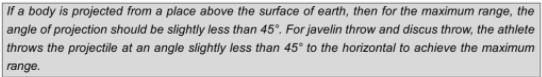(5) For height H to be maximum

$\mathrm{H}=\frac{\mathrm{u}^{2} \sin ^{2} \theta}{2 \mathrm{~g}}=\max \quad \text { i.e. } \sin ^{2} \theta=1(\max ) \text { or for } \theta=90^{\circ}$

So that $\mathrm{H}_{\max }=\frac{\mathrm{u}^{2}}{2 \mathrm{~g}} \quad$ When projected vertically (i.e. at $\left.\theta=90^{\circ}\right)$

in this case Range $R=\frac{u^{2} \sin \left(2 \times 90^{\circ}\right)}{9}=\frac{u^{2} \sin 180^{\circ}}{9}=0$

$\mathrm{H}_{\max }=\frac{\mathrm{u}^{2}}{2 \mathrm{~g}}$ (For vertical projection) and $\mathrm{R}_{\max }=\frac{\mathrm{u}^{2}}{\mathrm{~g}}$ (For oblique projection with same velocity)

so $\mathrm{H}_{\max }=\frac{R_{\max }}{2}$

If a person can throw a projectile to a maximum distance (with $\theta=45^{\circ}$ ) $R_{\max }=\frac{\mathbf{u}^{2}}{\mathrm{~g}}$.
.
The maximum height to which he can throw the projectile (with $\theta=90^{\circ}$ ) $\mathrm{H}_{\max }=\frac{R_{\text {max }}}{2}$

(6) At highest point

Potential energy will be max and equal to $(\mathrm{PE})_{4}=\mathrm{mgH}=\mathrm{mg} \cdot \frac{\mathrm{U}^{2} \sin ^{2} \theta}{2 \mathrm{~g}}$ or $(\mathrm{PE})_{\mathrm{H}}=\frac{1}{2} \mathrm{mu}^{2} \sin ^{2} \theta$

While K.E. will be minimum (but not zero) and at the highest point as the vertical component of velocity is zero.

$(\mathrm{KE})_{\mathrm{H}}=\frac{1}{2} \mathrm{mv}_{\mathrm{H}}^{2}=\frac{1}{2} \mathrm{~m}(\mathrm{u} \cos \theta)^{2}$

$=\frac{1}{2} \mathrm{mu}^{2} \cos ^{2} \theta$

so $(\mathrm{PE})_{\mathrm{H}}+(\mathrm{KE})_{\mathrm{H}}$

$=\frac{1}{2} m u^{2} \sin ^{2} \theta+\frac{1}{2} m u^{2} \cos ^{2} \theta$

= $\frac{1}{2} \mathrm{mu}^{2}=$ Total M.E.

So in projectile motion mechanical energy is conserved.

$\left(\frac{P E}{K E}\right)_{H}=\frac{\frac{1}{2} m u^{2} \sin ^{2} \theta}{\frac{1}{2} m u^{2} \cos ^{2} \theta}=\tan ^{2} \theta$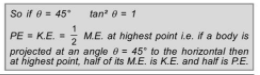(7) In case of projectile motion if range $R$ is $n$ times the maximum height $H,$ l.e. $R=n H$

then $\frac{u^{2} \sin 2 \theta}{g}=n \cdot \frac{u^{2} \sin ^{2} \theta}{2 g}$

or $2 \cos \theta=\frac{n \sin \theta}{2}$

or $\tan \theta=\frac{4}{n} \quad \Rightarrow \quad \theta=\tan ^{-1}\left(\frac{4}{n}\right)$

(8) Weight of a body in projectile motion is zero as it is a freely falling body.

So, that's all from this article. I hope you get the idea about the Oblique projectile motion. If you liked this explanation then please share it with your friends.